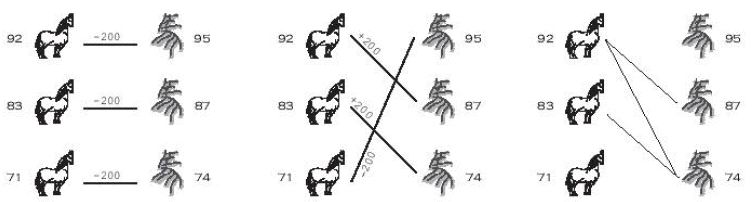## poj 2287

2019年02月28日 算法 ⁄ 共 3205字 ⁄ 字号 评论关闭
Tian Ji -- The Horse Racing
 Time Limit: 5000MS Memory Limit: 65536K Total Submissions: 9328 Accepted: 2866

Description

Here is a famous story in Chinese history.
That was about 2300 years ago. General Tian Ji was a high official in the country Qi. He likes to play horse racing with the king and others.

Both of Tian and the king have three horses in different classes, namely, regular, plus, and super. The rule is to have three rounds in a match; each of the horses must be used in one round. The winner of a single round takes two hundred silver dollars from
the loser.

Being the most powerful man in the country, the king has so nice horses that in each class his horse is better than Tian's. As a result, each time the king takes six hundred silver dollars from Tian.

Tian Ji was not happy about that, until he met Sun Bin, one of the most famous generals in Chinese history. Using a little trick due to Sun, Tian Ji brought home two hundred silver dollars and such a grace in the next match.

It was a rather simple trick. Using his regular class horse race against the super class from the king, they will certainly lose that round. But then his plus beat the king's regular, and his super beat the king's plus. What a simple trick. And how do you think
of Tian Ji, the high ranked official in China?Were Tian Ji lives in nowadays, he will certainly laugh at himself. Even more, were he sitting in the ACM contest right now, he may discover that the horse racing problem can be simply viewed as finding the maximum matching in a bipartite graph. Draw Tian's
horses on one side, and the king's horses on the other. Whenever one of Tian's horses can beat one from the king, we draw an edge between them, meaning we wish to establish this pair. Then, the problem of winning as many rounds as possible is just to find
the maximum matching in this graph. If there are ties, the problem becomes more complicated, he needs to assign weights 0, 1, or -1 to all the possible edges, and find a maximum weighted perfect matching...

However, the horse racing problem is a very special case of bipartite matching. The graph is decided by the speed of the horses -- a vertex of higher speed always beat a vertex of lower speed. In this case, the weighted bipartite matching algorithm is a too
advanced tool to deal with the problem.

In this problem, you are asked to write a program to solve this special case of matching problem.

Input

The input consists of up to 50 test cases. Each case starts with a positive integer n ( n<=1000) on the first line, which is the number of horses on each side. The next n integers on the second line are the speeds of Tian's horses. Then the next n integers
on the third line are the speeds of the king's horses. The input ends with a line that has a single `0' after the last test case.

Output

For each input case, output a line containing a single number, which is the maximum money Tian Ji will get, in silver dollars.

Sample Input

```3
92 83 71
95 87 74
2
20 20
20 20
2
20 19
22 18
0
```

Sample Output

```200
0
0
```

Source

dp啊，蛋疼死我了。

```#include<iostream>
#include<cstdio>
#include<algorithm>
#include<cstring>
using namespace std;
int n,a,b,f;
bool cmp(int a,int b){return a>b;}
int main()
{
while(scanf("%d",&n)&&n)
{
for(int i=0;i<n;i++)scanf("%d",&a[i]);
for(int i=0;i<n;i++)scanf("%d",&b[i]);
sort(a,a+n,cmp);
sort(b,b+n,cmp);
memset(f,0,sizeof(f));
for(int i=0;i<=n;i++)
{
f[i]=-i;
f[i]=-i;
}
for(int i=0;i<n;i++)
{
for(int j=0;j<n;j++)
{
f[i+1][j+1]=f[i][j+1];
if(a[i]>b[j])f[i+1][j+1]=max(f[i][j]+1,f[i+1][j+1]);
else if(a[i]==b[j])f[i+1][j+1]=max(f[i][j],f[i+1][j+1]);
}
}
/*for(int i=0;i<=n;i++)
{
for(int j=0;j<=n;j++)cout<<f[i][j]<<" ";
cout<<endl;
}*/
cout<<f[n][n]*200<<endl;
}
return 0;
}
```
【上篇】
【下篇】